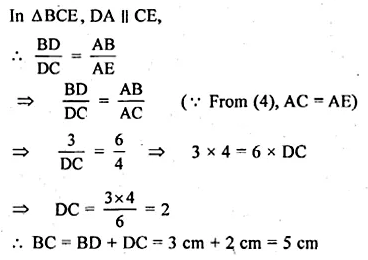# ML Aggarwal Class 10 Solutions for ICSE Maths Chapter 13 Similarity Ex 13.2

## ML Aggarwal Class 10 Solutions for ICSE Maths Chapter 13 Similarity Ex 13.2

ML Aggarwal Class 10 Solutions for ICSE Maths Chapter 13 Similarity Ex 13.2

Question 1.
(a) In the figure (i) given below if DE || BG, AD = 3 cm, BD = 4 cm and BC = 5 cm. Find (i) AE : EC (ii) DE.
(b) In the figure (ii) given below, PQ || AC, AP = 4 cm, PB = 6 cm and BC = 8 cm. Find CQ and BQ.
(c) In the figure (iii) given below, if XY || QR, PX = 1 cm, QX = 3 cm, YR = 4.5 cm and QR = 9 cm, find PY and XY.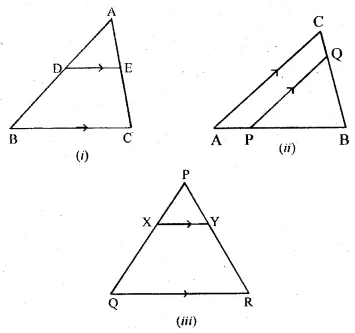Solution: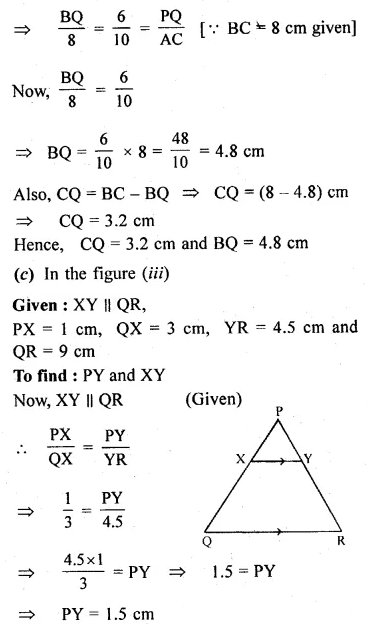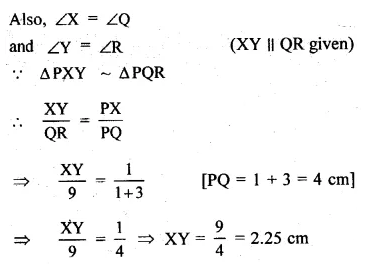Question 2.
In the given figure, DE || BC.
(i) If AD = x, DB = x – 2, AE = x + 2 and EC = x – 1, find the value of x.
(ii) If DB = x – 3, AB = 2x, EC = x – 2 and AC = 2x + 3, find the value of x.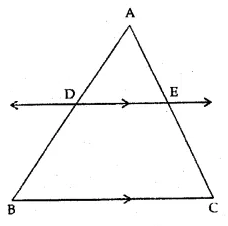Solution: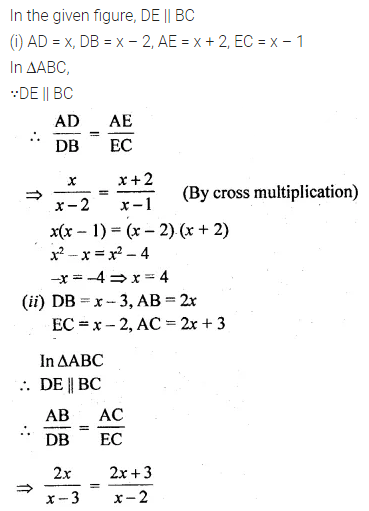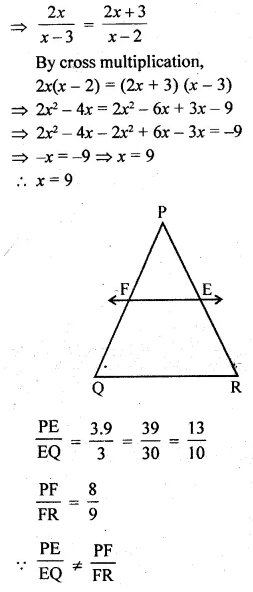Question 3.
E and F are points on the sides PQ and PR respectively of a ∆PQR. For each of the following cases, state whether EF || QR:
(i) PE = 3.9 cm, EQ = 3 cm, PF = 8 cm and RF = 9 cm.
(ii) PQ = 1.28 cm, PR = 2.56 cm, PE = 0.18 cm and PF = 0.36 cm.
Solution: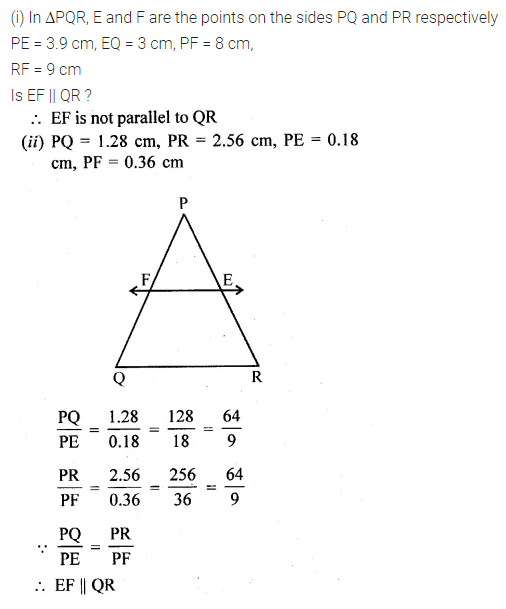Question 4.
A and B are respectively the points on the sides PQ and PR of a triangle PQR such that PQ = 12.5 cm, PA = 5 cm, BR = 6 cm and PB = 4 cm. Is AB || QR? Give reasons for your answer.
Solution: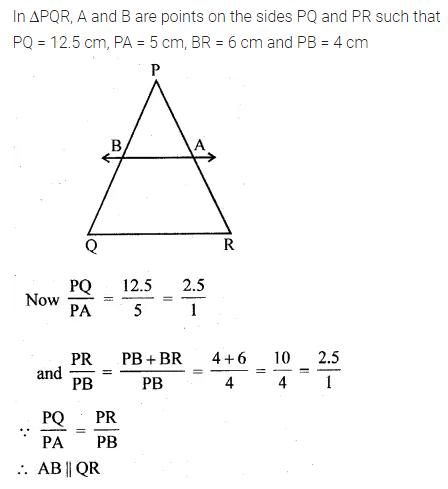Question 5.
(a) In figure (i) given below, DE || BC and BD = CE. Prove that ABC is an isosceles triangle.
(b) In figure (ii) given below, AB || DE and BD || EF. Prove that DC² = CF × AC.
Solution: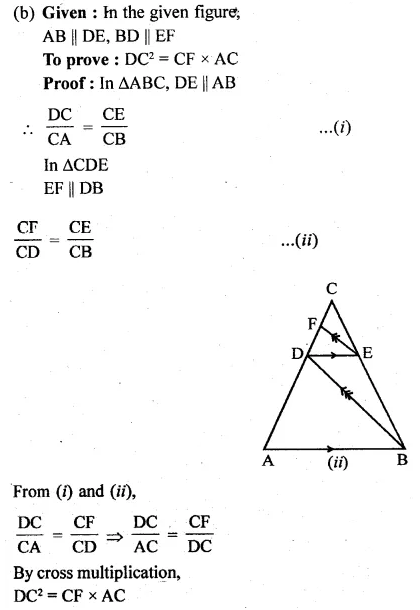Question 6.
(a) In the figure (i) given below, CD || LA and DE || AC. Find the length of CL if BE = 4 cm and EC = 2 cm.
(b) In the given figure, ∠D = ∠E and $$\frac { AD }{ BD } =\frac { AE }{ EC }$$. Prove that BAC is an isosceles triangle.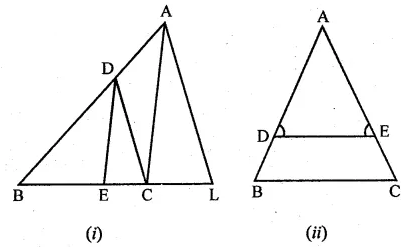Solution:Question 7.
In the figure given below, A, B and C are points on OP, OQ and OR respectively such that AB || PQ and AC || PR. show that BC || QR.Solution:Question 8.
ABCD is a trapezium in which AB || DC and its diagonals intersect each other at O. Using Basic Proportionality theorem, prove that $$\frac { AO }{ BO } =\frac { CO }{ DO }$$
Solution:Question 9.
(a) In the figure (1) given below, AB || CR and LM || QR.
(i) Prove that $$\frac { BM }{ MC } =\frac { AL }{ LQ }$$
(ii) Calculate LM : QR, given that BM : MC = 1 : 2.
(b) In the figure (2) given below AD is bisector of ∠BAC. If AB = 6 cm, AC = 4 cm and BD = 3cm, find BCSolution: